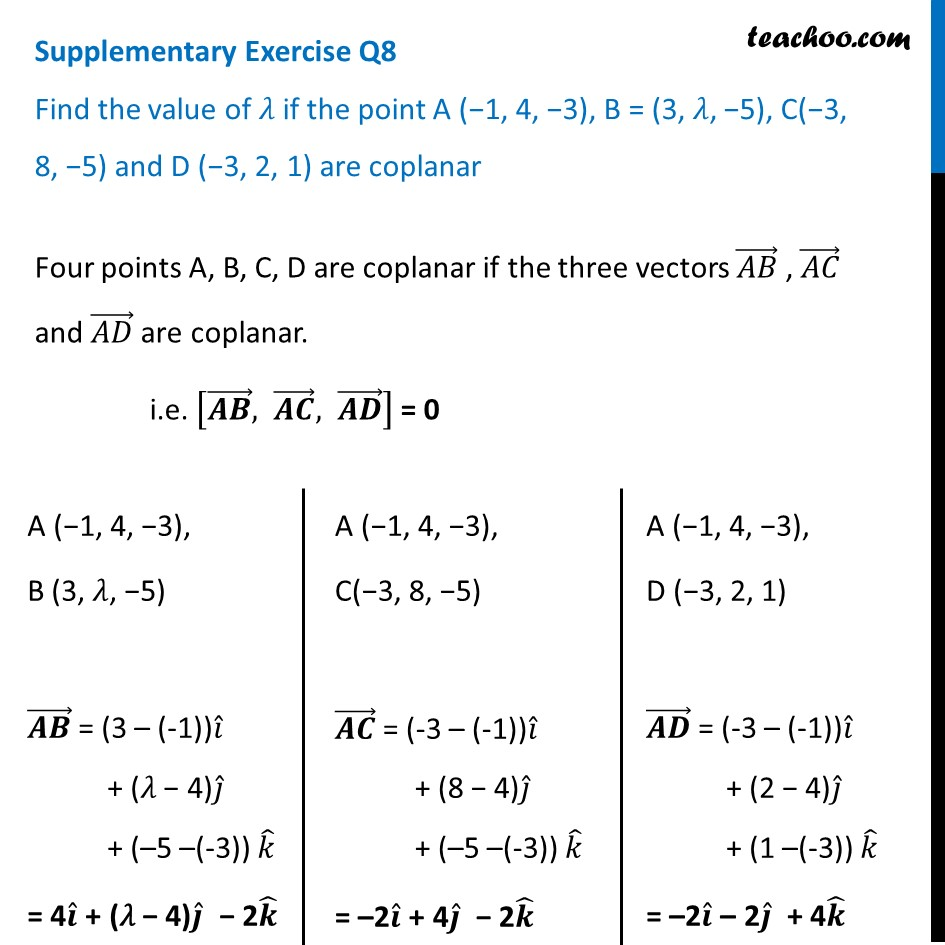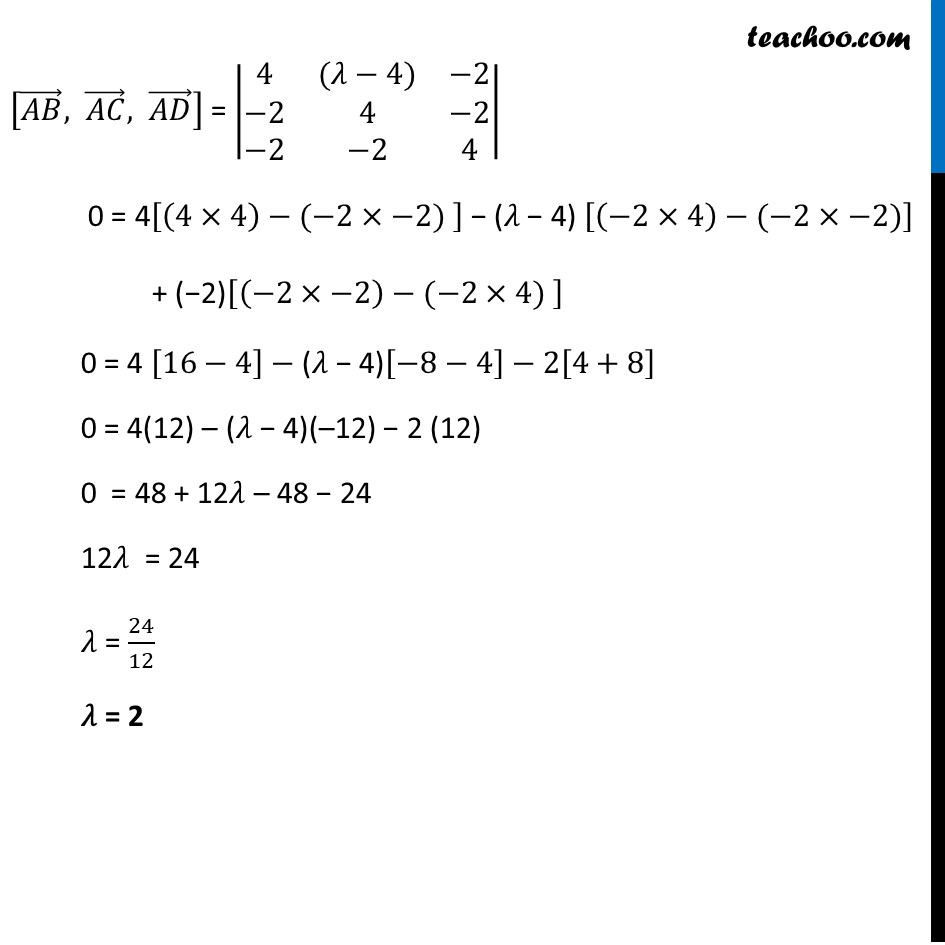Supplementary examples and questions from CBSE

Chapter 10 Class 12 Vector Algebra
Serial order wiseLearn in your speed, with individual attention - Teachoo Maths 1-on-1 Class

### Transcript

Supplementary Exercise Q8 Find the value of 𝜆 if the point A (−1, 4, −3), B = (3, 𝜆, −5), C(−3, 8, −5) and D (−3, 2, 1) are coplanar Four points A, B, C, D are coplanar if the three vectors (𝐴𝐵) ⃗ , (𝐴𝐶) ⃗ and (𝐴𝐷) ⃗ are coplanar. i.e. [(𝑨𝑩) ⃗, (𝑨𝑪) ⃗, (𝑨𝑫) ⃗ ] = 0 A (−1, 4, −3), B (3, 𝜆, −5) (𝑨𝑩) ⃗ = (3 – (-1))𝑖 ̂ + (𝜆 − 4)𝑗 ̂ + (–5 –(-3)) 𝑘 ̂ = 4𝒊 ̂ + (𝜆 − 4)𝒋 ̂ − 2𝒌 ̂ A (−1, 4, −3), C(−3, 8, −5) (𝑨𝑪) ⃗ = (-3 – (-1))𝑖 ̂ + (8 − 4)𝑗 ̂ + (–5 –(-3)) 𝑘 ̂ = –2𝒊 ̂ + 4𝒋 ̂ − 2𝒌 ̂ A (−1, 4, −3), D (−3, 2, 1) (𝑨𝑫) ⃗ = (-3 – (-1))𝑖 ̂ + (2 − 4)𝑗 ̂ + (1 –(-3)) 𝑘 ̂ = –2𝒊 ̂ – 2𝒋 ̂ + 4𝒌 ̂ [(𝐴𝐵) ⃗, (𝐴𝐶) ⃗, (𝐴𝐷) ⃗ ] = |■8(4&(𝜆−4)&−2@−2&4&−2@−2&−2&4)| 0 = 4[(4×4)−(−2×−2) ] − (𝜆 − 4) [(−2×4)−(−2×−2)] + (−2)[(−2×−2)−(−2×4) ] 0 = 4 [16−4]−"(𝜆 − 4)" [−8−4]−2[4+8] 0 = 4(12) – "(𝜆 − 4)"(–12) − 2 (12) 0 = 48 + 12"𝜆" – 48 − 24 12"𝜆" = 24 "𝜆" = 24/12 "𝜆" = 2